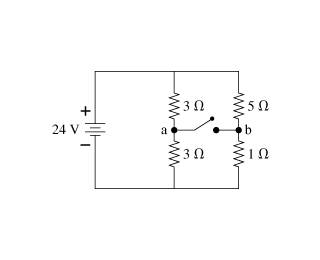# Potential difference of a battery

Linus Pauling
1. What is the potential difference DeltaVab between points a and b when the switch is open? Closed?2. DeltaV = IR

3. I solved I for the battery, which is 8A. What do I use for my R value to solve for DeltaV between a and b, though? I tried, for example, DeltaV = IR = 8A*6ohms = 48V. I guess I'm confused as to what I use for R to calculate the potential at each point, which are the values I use to get my answer. Could someone explain this to me conceptually? For the potential at A, for example, I tried multiplying the current (8A) by 3ohms because the current flows through that 3ohm resistor to get to point A...

## Answers and Replies

Homework Helper
What intermediate steps did you use , to get 8 Amp with the switch open ?
you considered R_left = 6 Ohm , and R_right = 6 Ohm ...
so : what's the voltage across R_left ? Voltage across R_right?
how much of this 8 Amp goes where ? (this charge is conserved, you know)

V = I R is a statement about ONE device .

Linus Pauling
3+3=6, 1+5=6. Then, inverse of [(1/6)+(1/6)] = 3. 24/3=8A.

It's correct.

The voltage across the bottom left resistor = the supply voltage times 3 divided by (the sum of the two series left resistors) .

So it is 24 * 3 / (3 + 3) volts.

Similarly,
The voltage across the bottom right resistor = the supply voltage times 1 divided by (the sum of the two series right resistors) .

So, it is 24 * 1 / (5 +1 ) volts.

Then, what is the voltage between the points a and b since the bottoms of these resistors are joined?

Linus Pauling
The voltage across the bottom left resistor = the supply voltage times 3 divided by (the sum of the two series left resistors) .

So it is 24 * 3 / (3 + 3) volts.

Similarly,
The voltage across the bottom right resistor = the supply voltage times 1 divided by (the sum of the two series right resistors) .

So, it is 24 * 1 / (5 +1 ) volts.

Then, what is the voltage between the points a and b since the bottoms of these resistors are joined?

Are you saying the voltage difference is 12V - 4V = 8V? Also, why do we care about the bottom left and right resistors specifically, since the current flows through the top two resistors to get to points a and b before reaching the bottom two resistors?

Are you saying the voltage difference is 12V - 4V = 8V? Also, why do we care about the bottom left and right resistors specifically, since the current flows through the top two resistors to get to points a and b before reaching the bottom two resistors?

Yes, that is right.

This is just two voltage dividers.

In each voltage divider the voltage across each lower resistor is equal to (the resistance of that resistor divided by the total resistance) times the supply voltage.

You could say the current in the left two resistors is 24 V / 6 ohms = 4 amps, so the voltage across the lower resistor is 4 amps * 3 ohms or 12 volts. (Ohm's Law)

Then the current in the right side is 24 volts / 6 ohms = 4 amps. So, the voltage across the 1 ohm resistor is 4 amps * 1 ohm or 4 volts.

So, the difference in voltage at a - b is 8 volts.
a is at +12 volts relative to the negative supply and b is at +4 volts relative to the negative supply.

The first method is much quicker, though.

You could read about voltage dividers here :
http://en.wikipedia.org/wiki/Voltage_divider

Linus Pauling
Thank you!

Now, what is deltaV between points a and b when the switch is closed? In this case, the top two (3 and 5 ohm) resistors are in parallel, as are the bottom two (3 and 1 ohm). The equivalent resistor for each of those pairs are in series with one another, so the current must be the same into and out of them. Solving I for the top two resistors:

[(1/3)+(1/5)]-1=1.875.
I = V/R = 24V / 1.875ohm = 12.8A

Then, V for point a: V = IR = (12.8A)(3ohm) = 38.4V

for point b: V = (12.8A)(1ohm) = 12.8V

deltaV = 25.6 V.... is this correct?

Also, in solving for a and b, I used the resistence of the bottom two resistors. If I use the two, I get a deltaV of the same magnitude but opposite sign (-25.6V). What does this mean?

EDIT: turns out 25.6V is incorrect....???